# Hassett 2: Weak approximation for function fields

Weak Approxmation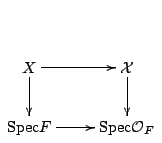where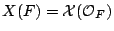,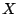smooth projective over a number field, and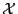an integral model of.

Definition. The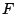-rational points ofsatisfy weak approximation if for each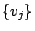a finite set of places, with completions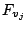, and open sets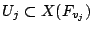, there exists an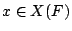with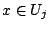for each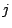.

Note that for nonarchimedean places,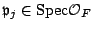,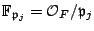, then we have reduction maps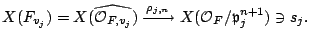The basic open subsets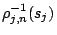have `fixed reduction modulo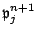'.

Remarks. By Hensel's lemma,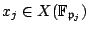gives a point in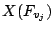if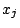is smooth.

Ifis regular, then ifcomes from a point in, thenis regular.

Function field analog

Now consider the diagram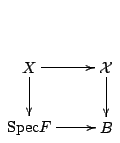where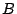is a smooth projective curve over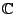,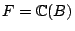, anda smooth projective variety overwith a regular projective model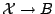. Fix a finite set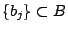,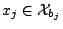smooth points, and local Taylor series data at these points,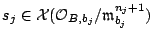,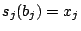.

Definition.satisfies weak approximation if for any such set of data there exists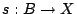so that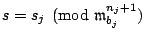.

Remarks.

1.satisfies weak approximation if and only if for each regular model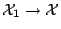, and pointsand smooth points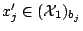, there exists a section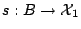with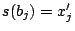.
2. If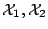are models of, then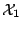satisfies weak approximation if and only if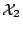does, so it makes sense to saysatisfies weak approximation.
3.-rational varieties satisfies weak approximation.

Rationally connected case

Let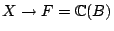be rationally connected, with model. Here we have the theorem:

Theorem. [Graber, Harris, Starr; Kollár] There exists a section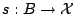. Choose pointssuch that the fibres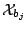are smooth, and choose points; then there exists a sectionwith.

This will not give Taylor series data, because once one blows up to get the second-order Taylor series, the fibres are no longer irreducible.

All the fibers ofare rationally chain connected, except for the degenerate fibers (e.g., reducible fibers), which might have to go through singular points. Also, for example, the cone over an elliptic curve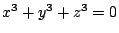is rationally chain connected but is not itself rationally connected.

Problem. Let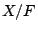be a smooth projective variety,,a curve. Ifis rationally connected, show thatsatisfies weak approximation.

Effectivity

Problem. Given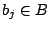,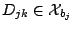of multiplicity one, does there exist an effective curve class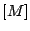such that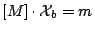, and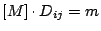.

Let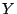be a projective smooth variety over. We have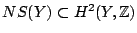, the Néron-Severi group, and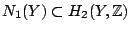, the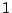-cycles. We have the cone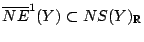, the cone of effective divisors; we also have the cone of moving curves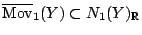, consisting of cycle classessuch that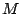is irreducible and passes through the generic point of.

Given an effective divisor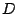and a moving class, then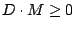.

Note that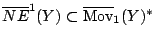, the dual cone.

Theorem. [Demailly, Peternell] Equality holds,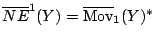.

As an application, this allows us to find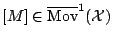with the desired intersection properties.

Back to the main index for Rational and integral points on higher dimensional varieties.